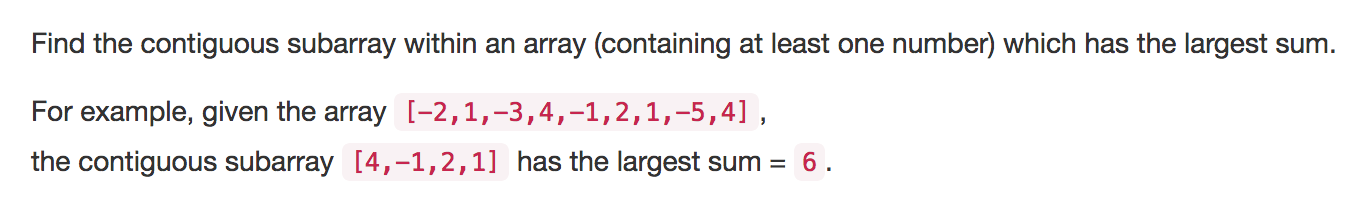# LeetCode#53暨最大连续子序列和问题class Solution {
public:
int maxSubArray(vector<int>& nums) {
//int length = nums.size();

int sum = 0;
int max = -2147483648;

for(vector<int>::iterator it1  = nums.begin(); it1 != nums.end(); it1++){
sum = *it1;
if(max < sum)
max = sum;

for (vector<int>::iterator it2 = it1+1; it2 != nums.end(); it2++) {
sum += *it2;

if(max < sum)
max = sum;
}
}

return max;
}
};

class Solution {
public:

int maxSubSumRec(vector<int>& nums, int left, int right){

if(left >= right){
return nums[left];
}

int i,center;
center = (left + right)/2;
int lmax = maxSubSumRec(nums, left, center - 1);
int rmax = maxSubSumRec(nums, center +1, right);
int mmax = nums[center], t = mmax;

for(i = center - 1; i >= left; --i){
t += nums[i];
mmax = max(mmax, t);
}

t = mmax;
for(i = center + 1; i <=right; ++i){
t += nums[i];
mmax = max(mmax, t);
}

return max(mmax, max(lmax, rmax));
}

int maxSubArray(vector<int>& nums) {

int length = int(nums.size()-1);
return maxSubSumRec(nums, 0, length);
}
};

class Solution {
public:

int maxSubArray(vector<int>& nums) {

vector<int>::iterator it  = nums.begin();
int maxSum = *it;
int theSum = *it;

for(it = it+1 ; it != nums.end(); it++){
theSum = max(theSum + *it, *it);

if(theSum > maxSum)
maxSum = theSum;
}

return maxSum;
}
};

#include <iostream>
#include <vector>

using namespace std;

int main(int argc, const char * argv[]) {

int n[] = {6,85,46,-74,26,30,25,-15,-22};

vector<int> a(n,n+9);

Solution sol;
cout<<sol.maxSubArray(a)<<endl;

return 0;
}

（本文完）

• # 波兰表达式（Reverse Polish Notation）（#150）

©️2019 CSDN 皮肤主题: 精致技术 设计师: CSDN官方博客